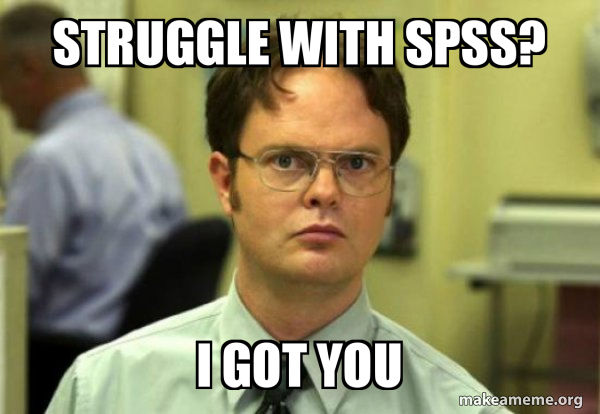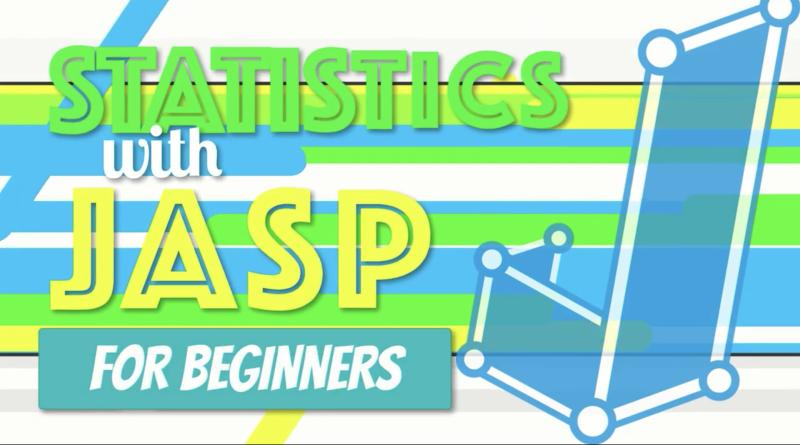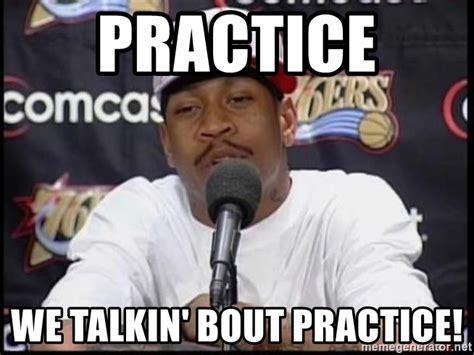# Statistics

## First Day Getting to Know You Survey

Establishing a rapport with students is connected to students’ success, interest, and pro-academic behaviors. Rapport can be particularly hard to establish in an online learning environment. One way to help establish rapport is by starting the semester with a survey that helps you get to know the students and their needs. Teachers who do this …## Video: One-Way ANOVA (SPSS for Beginners)

Video (11:19) from Research by Design described as follows, “Using the same example from the Wizard of Oz involving Munchkins and wicked witches in various regions that we used learning ANOVA by hand, we are going to learn about conducting a one-way ANOVA using SPSS. We will create the dataset in SPSS, conduct a one-way …## Video: Chi-Square (SPSS for Beginners)

Video (7:37) from Research by Design described as follows, “We are now going to learn how to calculate a one-way chi-square in SPSS. This is the House of Pigs Chi Square, using the dataset BuildingPermits.sav. A house inspector randomly samples building permits pulled by 70 little pigs who were building houses, to determine whether a …## Video: Independent-Samples t-test (SPSS for Beginners)

Video (8:11) from Research by Design and Dr. Todd Daniel, described as follows, “Another way of measuring the difference between two samples is to compare two unrelated groups or participants or samples. In this design, you measure two groups one time; in contrast, the previous paired test measured the same sample two times. With independent …## Video: One Sample and Paired-Samples t-test (SPSS for Beginners)

Video (6:02) from Research by Design and Dr. Todd Daniel, described as follows, “When we calculate the mean of a sample, we can then use a one-sample t test to compare that sample mean to another mean, such as a mean from a population, a normative group, or another known value (mean). The “one sample” …## Video: Correlation (SPSS for Beginners)

Video (9:52) from Research by Design and Dr. Todd Daniel, described as follows, “Using the dataset we created in the first video, we now explore the relationship between our two scale-level variables: height and weight. We will use Pearson’s correlation to measure this relationship. We will also learn about a point biserial correlation with gender.” …## Video: Frequencies and Descriptive Statistics (SPSS for Beginners)

Video (14:01) from Research by Design and Dr. Todd Daniel, described as follows, “Having learned how to create a variable, you are ready to begin entering data. Dr. Daniel begins with descriptive statistics (numbers that describe our data) and shows you how to do some basic data cleaning and data exploration with the Frequencies command …## Video: Introduction to SPSS & Creating Variables (SPSS for Beginners)

Video (10:15) from Research by Design and Dr. Todd Daniel, described as follows, “Are you ready to learn how to use SPSS for your introductory statistics class? You have come to the right place. This is the first in a series of eight videos that will introduce you to using SPSS for introductory statistics. This …## Video: How to Do Chi-Square in JASP

Video (10:27) from Research by Design in which, “We learn how to calculate a One-Way Chi-Square goodness of fit test in JASP using the setting for Multinomial Test. For the null hypothesis, we assume that the observed values in one group (the Pigs) do not differ from the values in a comparison population (the Bears) …## Video: How to Do Simple Linear Regression in JAS

Video (23:18) from Research by Design in which, “Exploring our data about burnout and job satisfaction, we predict an outcome with a single variable using simple linear regression in JASP. I explain how regression works, then open an SPSS dataset in JASP. We explore the assumptions of homoscedasticity and linearity with a scatterplot and normality …## Video: How to Do a Pearson Correlation in JASP

Video (18:47) from Research by Design in which, “Using a dataset about burnout and job satisfaction, we learn how to conduct a simple correlation, how to interpret it, and how to write it up in APA style. We begin by exploring the theoretical relationship among the variables to understand how correlation works. After we walk …## Video: How to Do an One-Way ANOVA in JASP

Video (14:02) from Research by Design in which, “We are off to see the wizard with an example of a one-way (factorial) ANOVA involving wicked witches and munchkins. We open a .CSV dataset in JASP, check the assumptions of homogeneity of variance and normality, conduct the test, interpret the results, and write up the findings …## Video: How to Do an Independent Samples t Test in JASP

Video (18:29) from Research by Design in which, “Using a dataset about puppy training, we learn how to set up and run an independent samples t test. We import data into JASP, conduct the test, interpret the results and write up the findings. We will learn to check the assumptions of homogeneity of variance using …## Video: How to Do a One Sample and Paired-Samples t Test in JASP

Video (16:57) from Research by Design in which, “The One Sample t-Test is a parametric procedure that tests whether a sample mean is statistically significantly different than a population mean or other known value. We will open a CSV dataset in JASP, check the assumptions of the test, conduct the one sample t-test, interpret the …## Video: Computing Central Tendency and Variability in JASP

Concise video (9:50) from Research by Design in which, “We walk through the steps to identify three measures of central tendency (mean, median, and mode) using JASP. This is an excellent introduction to JASP software and some of the functions that we will use throughout the introductory statistics course.” (7:41) “We will learn how to …## Video: Computing Frequencies and Creating an APA-Style Frequency Table Using JASP, Excel, and Word

Concise video from Research by Design in which, “We create an APA-style frequency table with scores in descending order, simple frequency, relative frequency, cumulative frequency, percentile, and sample size. We use JASP for the statistics, Excel for the formatting, and wrap it all up for presentation in Word. These same techniques work with open-source word …## Is One Study as Good as Three? College Graduates Seem to Think So, Even if They Took Statistics Classes

Abstract When people interpret the outcome of a research study, do they consider other relevant information such as prior research? In the current study, 251 college graduates read a single brief fictitious news article. The article summarized the findings of a study that found positive results for a new drug. Three versions of the article …## Who Needs Data? Publicly Available Datasets You Can Use in Class

Looking for large publicly available data for your students to explore? Below we provide a collection of sources and links. Title Description Link The Stanford Open Policing Project Standardized stop data are available to download (by location) from the table below. We provide these data in both CSV and RDS formats. Click here The General Social Survey (GSS) ​​​Since …## Practice: Repeated-Measures ANOVA

In this activity, students will take data from a fictitious design to practice conducting a Repeated-Measures Analysis of Variance (ANOVA). First, provide students with the research scenario and the accompanying questions to have them determine the research design, statistical analysis to use, and independent and dependent variables. Next, have students set up a data file …## Practice: Two-Way (Factorial) ANOVA

In this activity, students will take data from a fictitious 2 x 2 factorial design to practice conducting a Two-Way (Factorial) Analysis of Variance (ANOVA). First, provide students with the research scenario and the accompanying questions to have them determine the research design, statistical analysis to use, and independent and dependent variables. Next, have students …